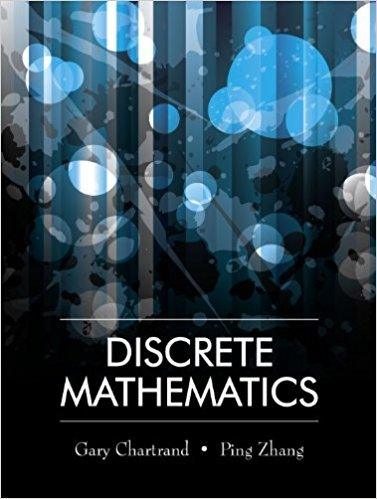×
×

# A total of 10 prizes of equal value are to be given to 10 graduating seniors. A total ofISBN: 9781577667308 385

## Solution for problem 1 Chapter 8.5

Discrete Mathematics | 1st Edition

• Textbook Solutions
• 2901 Step-by-step solutions solved by professors and subject experts
• Get 24/7 help from StudySoup virtual teaching assistantsDiscrete Mathematics | 1st Edition

4 5 1 419 Reviews
24
5
Problem 1

A total of 10 prizes of equal value are to be given to 10 graduating seniors. A total of 12 seniors are eligible for these prizes. In how many ways can these prizes be distributed?

Step-by-Step Solution:
Step 1 of 3

--- --f - - --- -t-C.r 11DPOil~ ~ [Xi),v.eiet~J 11iJo~~ -s_ --- --_1-welto~DFcethterultdJ >ca.t/r--' '----------· (P1'i1UlODS_...

Step 2 of 3

Step 3 of 3

##### ISBN: 9781577667308

The full step-by-step solution to problem: 1 from chapter: 8.5 was answered by , our top Math solution expert on 03/13/18, 07:11PM. Since the solution to 1 from 8.5 chapter was answered, more than 210 students have viewed the full step-by-step answer. The answer to “A total of 10 prizes of equal value are to be given to 10 graduating seniors. A total of 12 seniors are eligible for these prizes. In how many ways can these prizes be distributed?” is broken down into a number of easy to follow steps, and 35 words. Discrete Mathematics was written by and is associated to the ISBN: 9781577667308. This full solution covers the following key subjects: . This expansive textbook survival guide covers 79 chapters, and 1714 solutions. This textbook survival guide was created for the textbook: Discrete Mathematics, edition: 1.

Unlock Textbook Solution